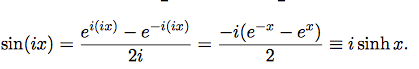# Sinh / sin

## Homework Statement

So this is a really simple problem and i know I'm missing something really obvious but i just can't spot it.## The Attempt at a Solution

so in the second part above I get :
e-x - ex/2i. However I don't get the next bit as the 2i on the denominator is now factored into the top equation to give -ie-x + iex/2. I don't get how the 2 equations are equivalent and what they've done to get the i from the denominator to the numerator. If anyone could explain how this happened that'd be great!

fresh_42
Mentor
2021 Award
What is i?

Do you know what ##1/i## is?

Do you know what ##1/i## is?
i-1?

fresh_42
Mentor
2021 Award
i-1?
First answer my question and then use it to answer struggles'. ##i^{-1}## is simply another form of ##\frac{1}{i}## which doesn't really help.

robphy
Homework Helper
Gold Member
Suppose you didn't know what ##i## is
but it seems that (from your second equals sign) ##\frac {1}{i}= -i##... what must ##i## satisfy?

fresh_42
Mentor
2021 Award
Suppose you didn't know what ##i## is
but it seems that (from your second equals sign) ##\frac {1}{i}= -i##... what must ##i## satisfy?
How is it defined? What stands ##i## for? There must have been some explanation for it.
Remark: Sorry, for confusing your names, struggles and axmls!

Though it isn't obvious that this is the way to find this, here's a hint:
$$\frac{1}{i} = \frac{1}{i} \frac{i}{i}$$

so i = √-1 so i2 = -1. so 1/i must equal 1/(-1)1/2 = (-1)-1/2? is that what you were getting at fresh-_42?
So axmls 1/i = i/i^2 = -i! Thanks both of you!